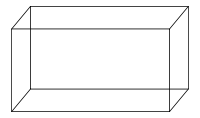# Find The Dimensions Of This Cuboid

Geometry Level 1Find the sum of length, breadth & height of a cuboid whose whole surface area is 214 $\text{cm}^2\negthickspace,\,$ with a base area of 42 $\text{cm}^2\negthickspace,\,$ and with volume 210 $\text{cm}^3\negthickspace.$

×